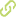# Sorption isotherms of mango (Mangifera Indica L.) pulp freeze-dried•
• Overview
•
•
• View All
•

### Abstract

• The equilibrium moisture contents of mango pulp freeze- dried was determined using the gravimetric - static method at 288.15, 298.15 y 308.15 K for water activity (aw) ranging from 0.11 to 0.89. At a given aw, the results showed that the moisture content decreases with increasing temperature. Mathematical models were evaluated to determine the best fit for the experimental data. A nonlinear regression-analysis method was used to evaluate the constants of equations. The BET and GAB models were the most suitable to describe the sorption curves; the monolayer-content values for the sorption at different temperatures were calculated.

### Publication date

• December 1, 2010